# 配色的用户体验——颜色对比度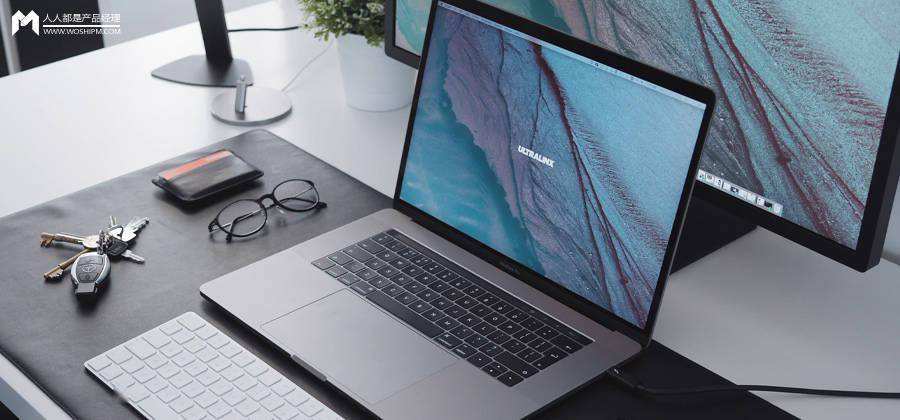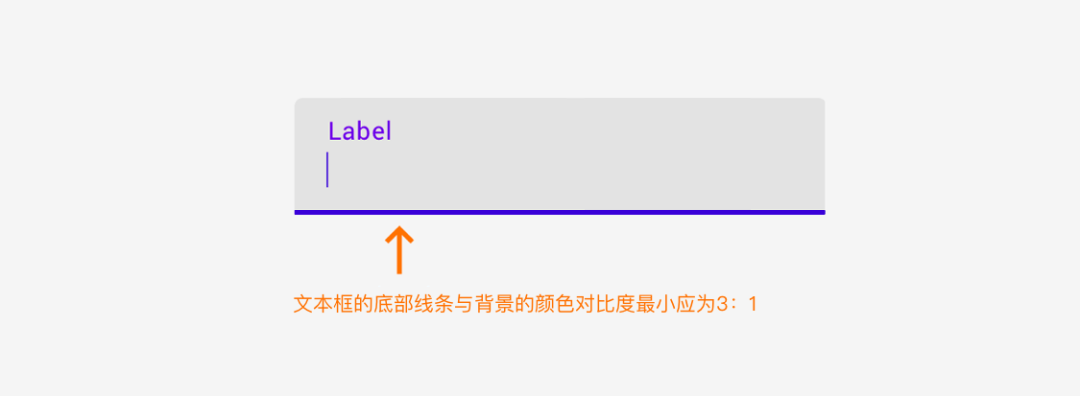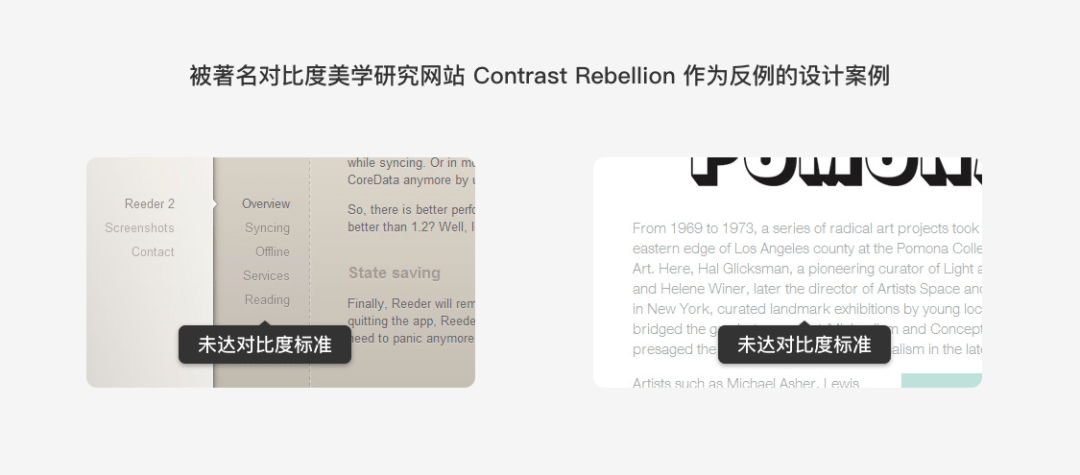WCAG颜色对比度标准定义的目的是让文本和背景之间存在足够的对比度，确保绝大范围视力程度的人群都易于阅读；也就是说，符合WCAG该标准的文字或图像，将有足够高的色彩对比度，使之很容易地从背景中被辨识出来。

WCAG制定了两条标准条例，分别是「1.4.3条例：对比度（最小）标准」（即AA标准）和「1.4.6条例：对比度（加强）标准」（即AAA标准）；AAA标准比AA标准要更加严苛，适合视觉要求更严格的产品类型。

• 1.4.3 对比度（最小）: 普通文本的视觉呈现与背景至少要有4.5:1的对比度，大文本¹与背景至少有3:1的对比度。
• 1.4.6 对比度（加强）: 普通文本的视觉呈现与背景至少有7:1的对比度，大文本¹与背景至少有 4.5:1的对比度。

（温馨提示：数学不好的朋友…可以直接跳到下一节使用便捷工具…）

XsRGB 在此指代 RsRGB, GsRGB, 或 BsRGB，取值为 XsRGB = X8bit/255 「X8bit 指R、G、B通道各自在8位/通道下 0-255 的取值」。

• 对于黑色：RsRGB = GsRGB = BsRGB = 0/255 = 0
• 对于白色：RsRGB = GsRGB = BsRGB = 255/255 = 1
• 黑色：RsRGB = GsRGB = BsRGB = 0 ＜ 0.03928
• 黑色：R = G = B = 0/12.92 = 0
• 白色：RsRGB = GsRGB = BsRGB = 1 ＞ 0.03928
• 白色：R = G = B = [(1+0.055)/1.055]^2.4 = 1
• 黑色的相对亮度 L1 = 0.2126 * 0 + 0.7152 * 0 + 0.0722 * 0 = 0
• 白色的相对亮度 L2 = 0.2126 * 1 + 0.7152 * 1 + 0.0722 * 1 = 1
• 黑色文本与白色背景的对比度 = (0+0.05) / (1+0.05) = 1:21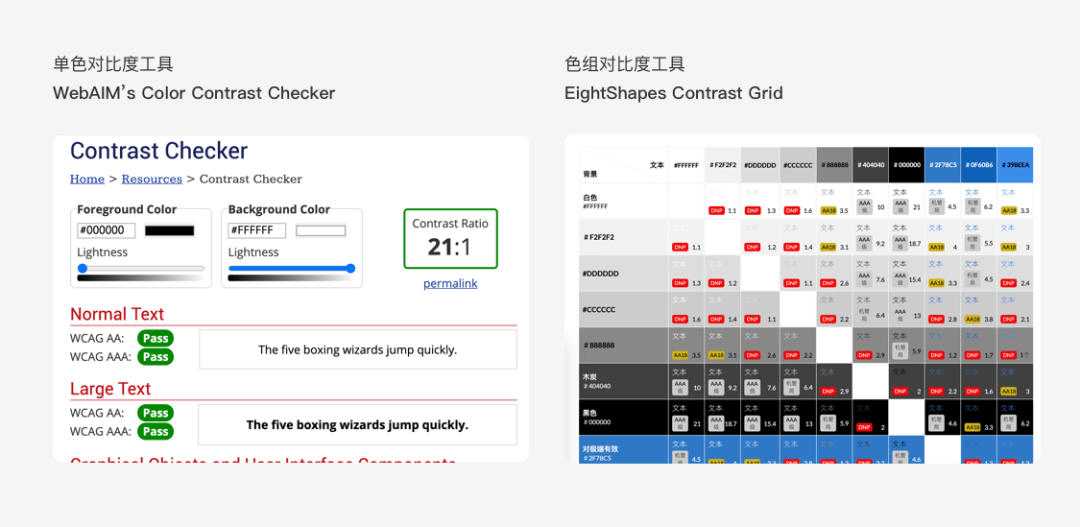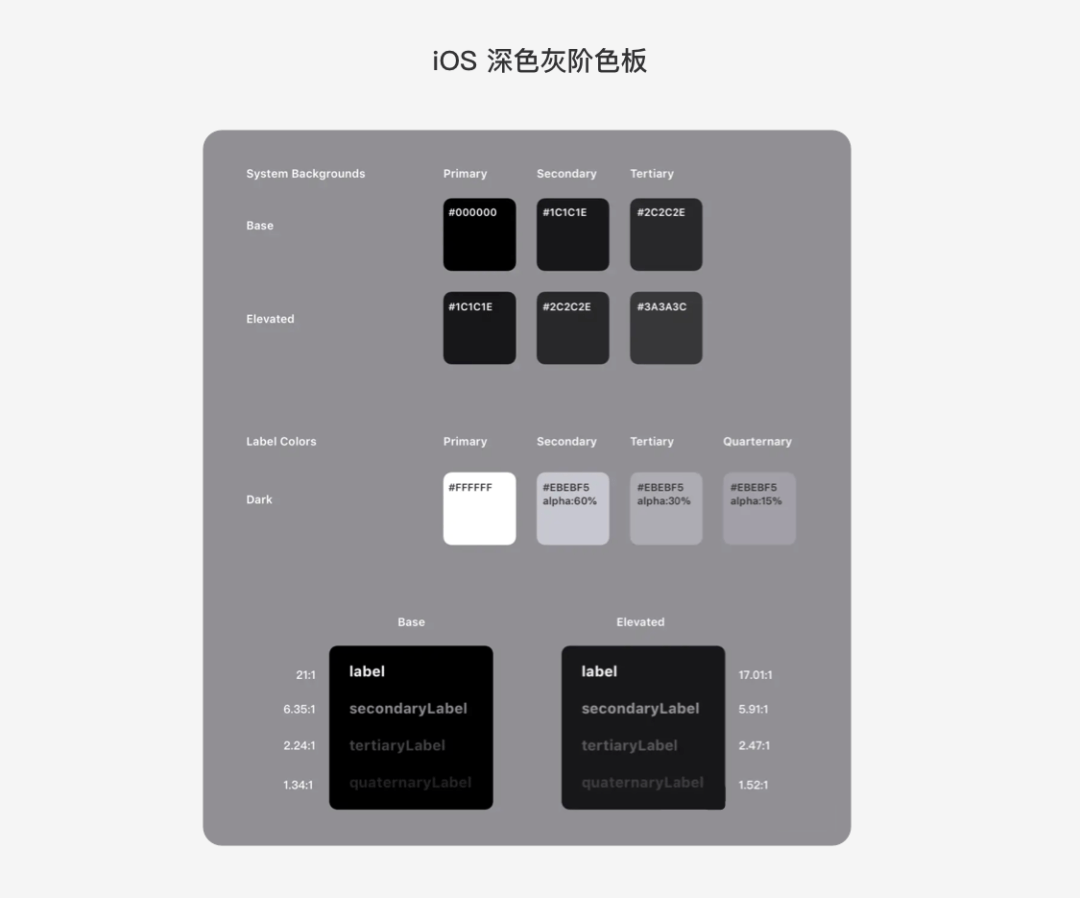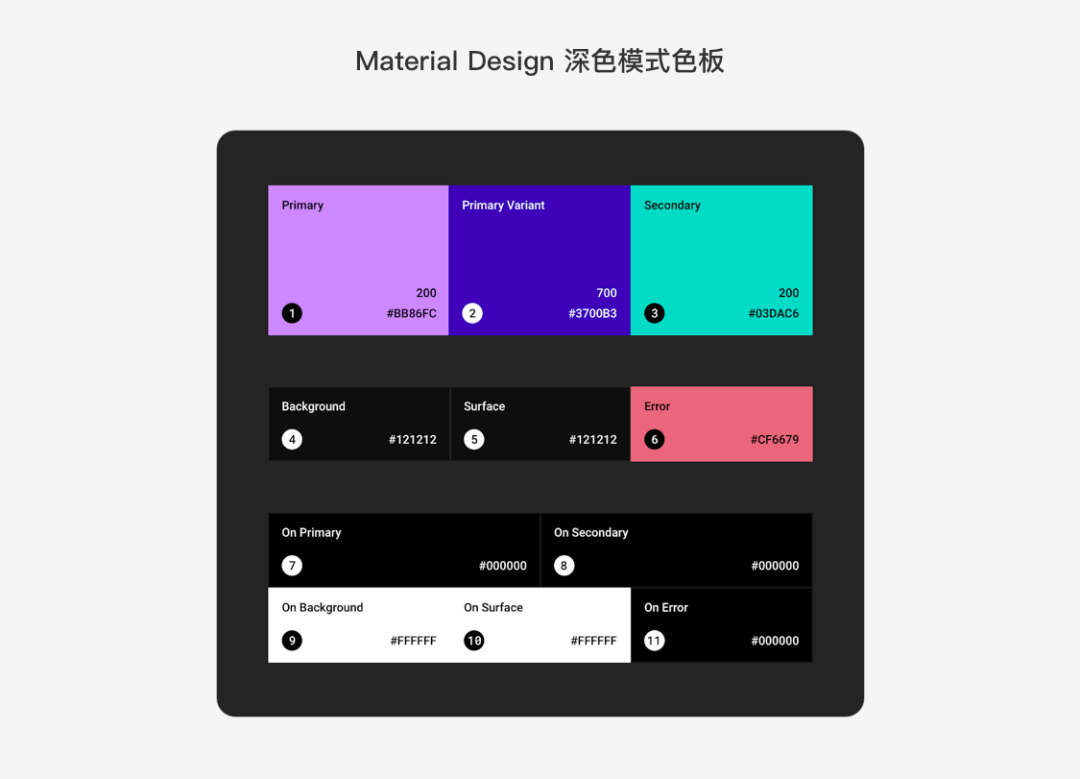#专栏作家#

UCD耍家，公众号：UCD耍家（ID：ucdplayer），人人都是产品经理专栏作家。